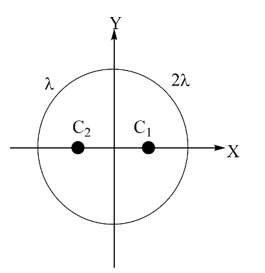Methods to locate centre of mass
Question

# Two semicircular rings of linear mass density  $\lambda$ and  $2\lambda$ and of radius R are joined to form a complete ring. The distance of the center of mass of the complete ring from its centre is

Easy
Solution

##${\mathrm{C}}_{1}\left(\frac{2\mathrm{R}}{\mathrm{\pi }},0\right)$${\mathrm{C}}_{2}\left(-\frac{2\mathrm{R}}{\mathrm{\pi }},0\right)$X – Co-ordinate of COM of the system $=\frac{\mathrm{m}\left(-2\mathrm{R}/\mathrm{\pi }\right)+2\mathrm{m}\left(2\mathrm{R}/\mathrm{\pi }\right)}{3\mathrm{m}}=\frac{2\mathrm{R}}{3\mathrm{\pi }}$

Get Instant Solutions